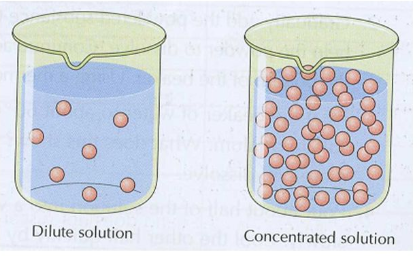# Acids Bases and Salts

## Properties of Acids, Bases and Salts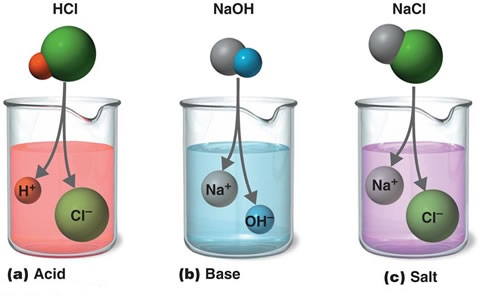Acids, bases and salts affect chemistry as well as our day to day life. They can be easily identified by their taste; that is acids taste sour and bases taste bitter and salts itself have salty taste.Sour taste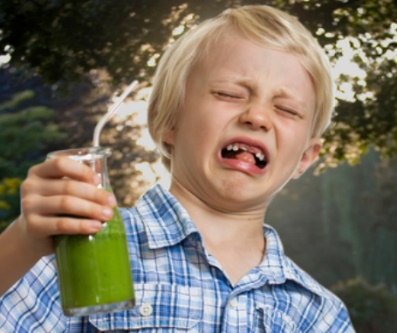Bitter taste

Acids are usually found in many substances including various food items but their presence in many fruits is very prominent, for example: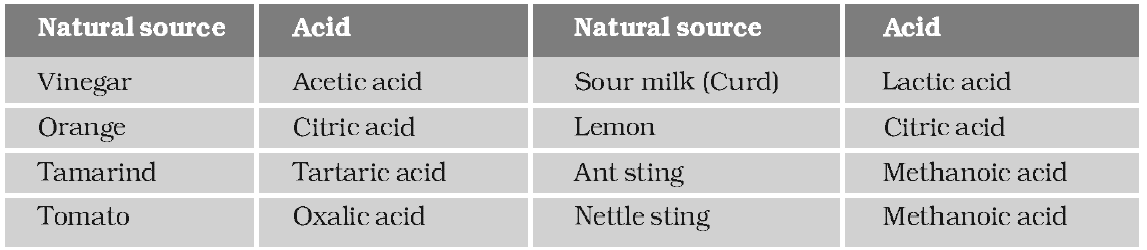Apart from these, there are some acids which are widely used in the laboratory, like the hydrochloric acid, sulphuric acid and nitric acid.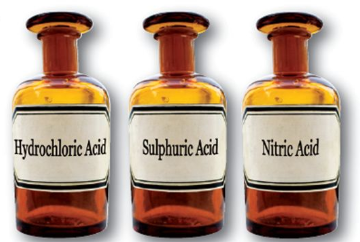Usually bases are found in household cleaners only to clean grease from the windows and the floors and it is also found in soaps, toothpaste, egg whites, dish washing liquids and household ammonia.Our body contains some very common acids in the stomach like the dilute hydrochloric acid, which causes food indigestion. When the contents of our stomach become too acidic, we usually get indigestion and a burning sensation in our stomach.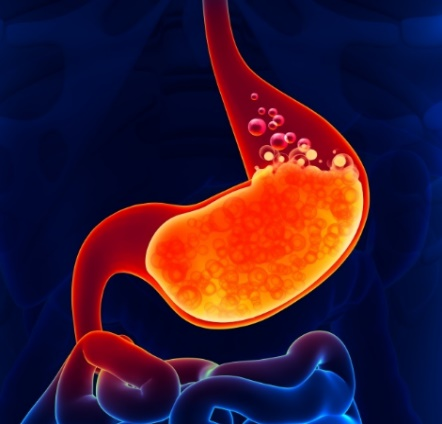Acidity in stomach

Acids and bases also regulate some metabolic activities in the human body through the process of equilibrium. Bee stings are acidic in nature while the wasp stings are alkaline in nature.

All acids when react with metals generate hydrogen gas. Hydrogen is usually common to all acids.

${\text{Acid + Metal = Salt + Hydrogen}}$

Properties of acids

• • Acid is a compound which yields hydrogen ion $({H^ + })$, when dissolved in water.

•              $HCl + {H_2}O \to {H^ + } + C{l^ - }$

• • Acid is sour to the taste and it is corrosive in nature. The pH of acids is less than 7.

•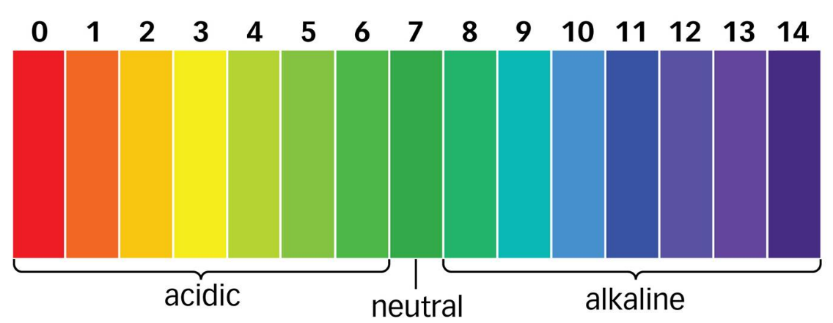• • All acids react with metal to release hydrogen gas. For example, zinc metal reacts with hydrochloric acid to form zinc chloride and hydrogen gas.

•                  $Zn + 2HCl \to ZnC{l_2} + {H_2}$

• • When acids react with limestone (CaCO3), it produces carbon dioxide. For example, $HCl$ reacts with limestone to produce carbonic acid and calcium chloride.

•                 $CaC{O_3} + 2HCl \to CaC{l_2} + C{O_2} + {H_2}O$

• • Acids are classified into organic and inorganic acids. The best example of organic acid is acetic acid $(C{H_3}COOH)$, and inorganic acids are those which are produced from minerals; for example, sulphuric acid $({H_2}S{O_4})$, and hydrochloric acid , etc.

• • Acid usually converts blue litmus paper to red litmus paper.

•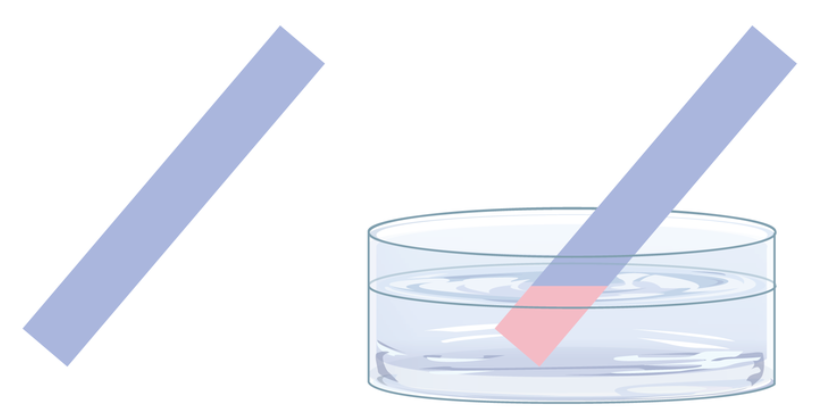• • Acids have a tendency to quickly corrode the metal surfaces.

•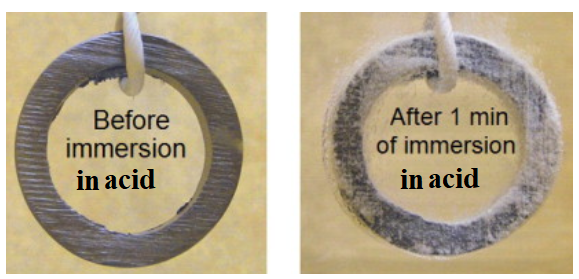On the basis of number of hydrogen ion, acids can be classified as: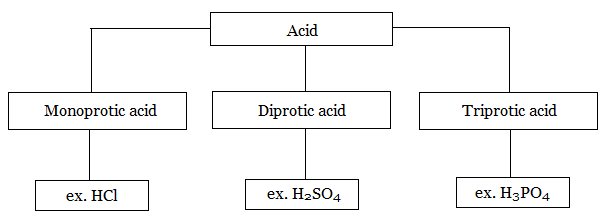1.Monoprotic acid – They can produce one mole of ${H^ + }$ions per mole of acid, $ex - HCl$.

2.Diprotic acid – They can produce two moles of ${H^ + }$ions per mole of acid, $ex - {H_2}S{O_4}$.

3.Triprotic acid – They produce three moles of ${H^ + }$ions per mole of acid, $ex - {H_3}P{O_4}$.

On the basis of strengths to donate hydrogen ions, acids can be classified as: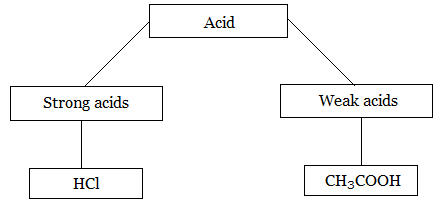Strong acids: These acids get completely (100%) ionized in the aqueous solutions. Thus, at equilibrium, the concentration of acid molecules becomes very less and concentration of hydrogen ion reaches to the maximum; for example, $HCl,\;HN{O_3},\;HCl{O_4}$.

Weak acids: These acids are only partially ionized in solution at equilibrium state. At equilibrium state, molecules of acid are present in a considerable amount and the concentration of hydrogen ion is less, for example, $HF,\;C{H_3}COOH$.

Properties of bases:

• • Bases are compounds which yields hydroxide ion $(O{H^ - })$, when it is dissolved in water.

•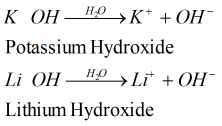• • Bases are bitter in taste and are corrosive in nature. They usually feel slippery and very soapy.

• • Bases are good conductor of electricity and usually shows a pH value of more than 7.

•• • Bases react with oils and grease to form molecules of soap.

• • Bases convert red litmus paper to blue litmus paper.

•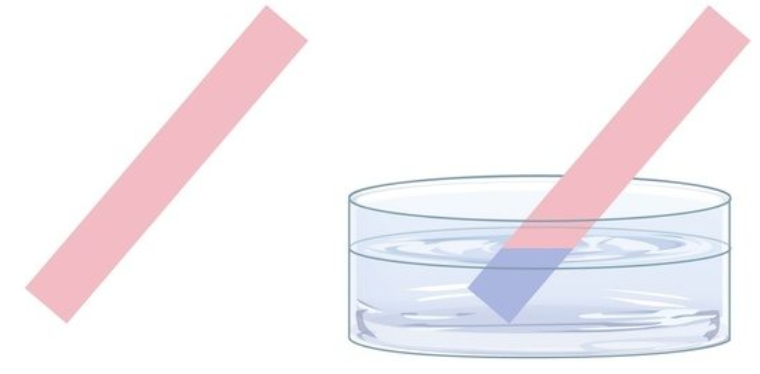• • Bases also have the tendency to corrode the surface of metals.

• Strength of bases-

Strong bases: These are the bases which are completely ionized in water to produce hydroxide ions, e.g., sodium hydroxide

$NaO{H_{(s)}} \rightleftharpoons Na_{(aq)}^ + + OH_{(aq)}^ -$

Weak bases: These are the bases which are partially ionized and the equilibrium lies mostly towards the reactants side, e.g., ammonia in water

$N{H_{3(aq)}} + {H_2}{O_{(l)}} \rightleftharpoons N{H_4}_{(aq)}^ + + OH_{(aq)}^ -$

Properties of salts: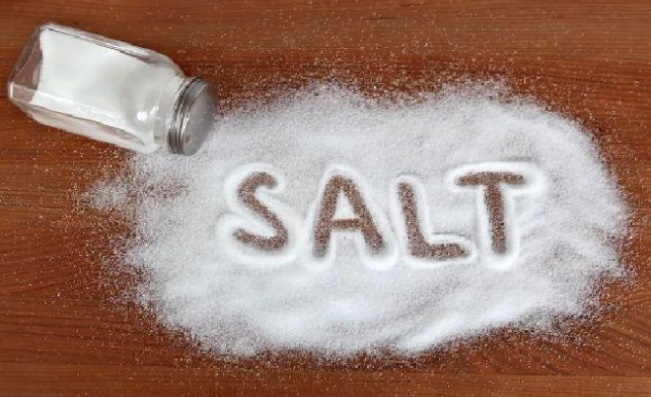Salts are formed by the combination of acid and base through the neutralization reaction.

The acidic and basic nature of salts usually depends on the acid and base from which the salt evolved in neutralization reaction.

Example:

$NaOH + HCl \to NaCl + {H_2}O$
$HCl{\text{ }} + {\text{ }}N{H_4}OH{\text{ }} \to {\text{ }}N{H_4}Cl{\text{ }} + {\text{ }}{H_2}O$
$C{H_3}COOH{\text{ }} + {\text{ }}NaOH{\text{ }} \to {\text{ }}C{H_3}COONa{\text{ }} + {\text{ }}{H_2}O$
$C{H_3}COOH{\text{ }} + {\text{ }}N{H_4}OH{\text{ }} \to {\text{ }}C{H_3}COON{H_4} + {\text{ }}{H_2}O$

The most well-known or common salt is sodium chloride or table salt which is formed by the combination of a strong base sodium hydroxide and strong acid hydrochloric acid.

$HC{l_{(aq)}} + NaO{H_{(aq)}} \to NaC{l_{(aq)}} + {H_2}{O_{(l)}}$

Other examples include Epsom salt $(MgS{O_4})$ which is used in bath salts, ammonium nitrate $(N{H_4}N{O_3})$ is used as fertilizer, and baking soda $(NaHC{O_3})$ is used in cooking.

The pH of a solution of salt also depends on the strength of acids and bases which are combined in the neutralization reaction.

Addition of Acids or Bases to Water:

The process of dissolving an acid or a base in water is highly exothermic. As this reaction usually generates a lot of heat, so there must be exclusive care taken while mixing the concentrated acids with water, especially when nitric acid or sulphuric acid is mixed with water.

Rules: The acid must be added slowly to the water with continuous and constant stirring, otherwise it can cause the mixture to splash out which in turn cause burns.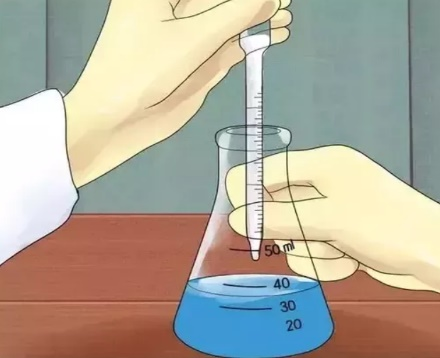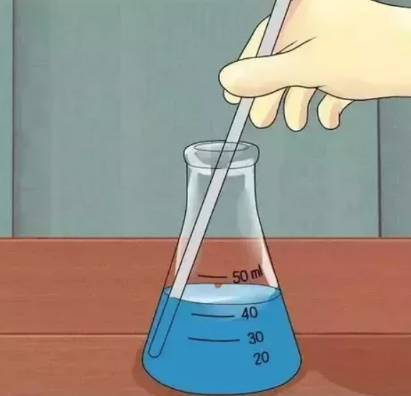The glass container may also can break due to excessive heating which can cause damage. When an acid or base is mixed with water, it results in dilution. It decreases the concentration of ions $({H_3}{O^ + }/O{H^ - })$ per unit volume thereby easily dissipating the effect of heat.